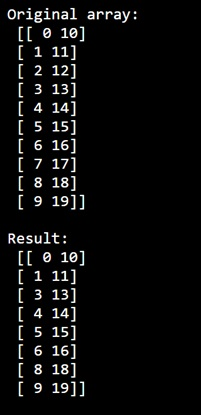# How to delete a batch of rows of a NumPy array simultaneously?

Learn, how to delete a batch of rows of a NumPy array simultaneously in Python?
Submitted by Pranit Sharma, on December 30, 2022

NumPy is an abbreviated form of Numerical Python. It is used for different types of scientific operations in python. Numpy is a vast library in python which is used for almost every kind of scientific or mathematical operation. It is itself an array which is a collection of various methods and functions for processing the arrays.

## Deleting a batch of rows of a NumPy array simultaneously

Suppose we are given with a multi-dimensional array with 10 rows and we need to delete some specific rows from this array (say 3rd or 7th row).

For this purpose, we will use numpy.delete() method and pass an argument "axis=0". This method returns a new array with sub-arrays along an axis deleted.

To delete some specific elements from an array, we will pass the list of indices of those rows which we want to remove and pass an argument "axis = 0".

Let us understand with the help of an example,

## Python code to delete a batch of rows of a NumPy array simultaneously

```# Import numpy
import numpy as np

# Import pandas
import pandas as pd

# Creating a numpy array
arr = np.arange(20).reshape(10,2, order='F')

# Display original array
print("Original array:\n",arr,"\n")

# Defining a list of indices for specific rows
ind = [2,7]

# Deleting the rows
res = np.delete(arr, ind , axis=0)

# Display result
print("Result:\n",res,"\n")
```

Output: